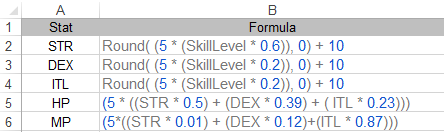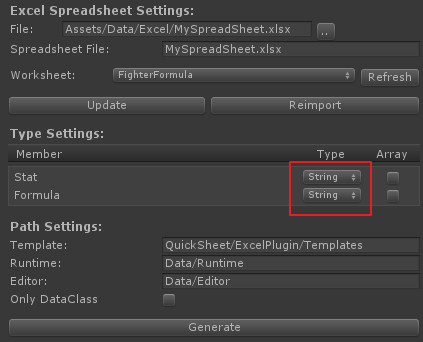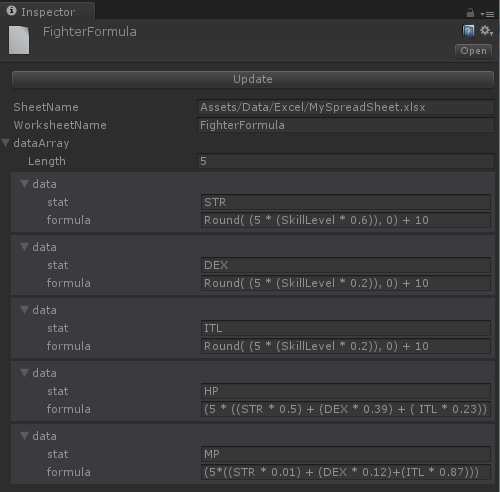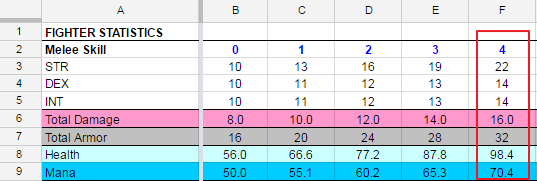# Automation of Formula Calculation

Characters in a RPG normally have various stats such as HP, MP, level etc. and those are usually calculated via various given formulas. For an example, power of a character is increased as they level up and this can be represented with a form of formula.

But there is a problem how the formulas are dealt with. You can write the formulas within code. Easy but it has a problem that it should be rewritten everytime whenever the formula is changed. It is not a surprise thing a game designer changes that again and again over the whole development time.

So we need more flexible way to deal with the formulas as possible as a form of data not a code piece.

This section of the document describes how the formula calculations can be easily done with a spreadsheet and Unity-Quicksheet plugin by fully data-driven way.

Before futher reading, if you're not familiar with typical stats and formulas of a RPG, highly recommended to see the article, The craft of game systems: Practical examples first.

First thing to do is defining various stats and formulas on a spreadsheet, so it can make to be used in the game .

You can use either of Excel or Google Spreadsheet whatever you prefer but the description on this document is based on Excel spreadsheet.

Let's consider that a character, in the game you try to make, increases in power as he levels up. He has three basic stats to describe his power. Each of that are strength(STR), dexterity(DEX) and intelligence(ITL). As his stats increase, he get more health(HP) and mana(MP).

The following image shows each of stat and its corresponding formula on the spreadsheet.The data on the spreadsheet will be used for the example on this document.

Note that `Stat` and `Formula` of the above image are borrowed from the article, The craft of game systems: Practical examples.

## Data Importing

First, let's import all data in the worksheet into Unity editor.

Create import setting asset file and import the file by specifing the spreadsheet. (See the How to work with Excel page for the detail steps.)

Set both type of `Stat` and `Formula` in the 'Type Settings' as string type. `Stat` is just name of each stat which are belong to a player so set its type as string seems to be ok but why `Formula` is also set as string? The answer will be found later. At the moment, just think it again and you will get to know that there is no ohter proper type for the formula else than string.Click `Generate` button which will generate all necessary scirpt files under the directories specified on the 'Editor' and 'Runtime' textfield.

Then select .xlsx Excel file in the Project window and select `Reimport` menu shown by right-click of the selected excel file.

As reimporting the excel file, it creates 'ScriptableObject' as an asset file with the name of worksheet and imports all data into it. Select the created asset file and check the improted data is correct. You may see the following image on the Inspector view of Unity editor:You can see the generated code, especially FighterFormuladata class which can be found under the directory specified `Runtime` setting.

``````[System.Serializable]
{
[SerializeField]
string stat;
public string Stat { get {return stat; } set { stat = value;} }

[SerializeField]
string formula;
public string Formula { get {return formula; } set { formula = value;} }
}
``````

If you're not familar to import data from a spreadsheet, see How to work with Excel or How to work with Google Spreadsheet page depends on the type of your spreadsheet.

## Writing Calculation Code

Now, let's write down code to calculate each of stat with the given formula imported from the spreadsheet.

First of all, we need a class to represent all stats of a fighter type of player in the game. The following simple POCO class, `PlayerStat` can be used for that. It is nothing else but has all stat which are shown on the spreadsheet as properties except `SkillLevel` property.

``````public class PlayerStat
{
public int SkillLevel { get; set; }
public float STR { get; set; }
public float DEX { get; set; }
public float ITL { get; set; }
public float HP { get; set; }
public float MP { get; set; }
}
``````

Next thing, we need to do is doing calculation each of stat with its correspond formula and set the result back to each of stat.

Typically, a formula of a RPG is just a polynomial and the imported formulas of ours are string data. So we need a calculator can calculate a polynomial as form of string data.

And so, how do we can calculate the formula as form of string data?

Though it can be done with writing a simple calculator but luckily there are already bunch of calculators written by C# exist. A matter of course, we don't need to reinvent the wheel.

One of notable thing from those is A Calculation Engine for .NET which is simple to use and already has most functionalities for our formula calculation.

Also there is Zirpl CalcEngine, a port of A Calculation Engine for .NET to a portable .NET library(PCL) which can be found on this github project page.

Let's see how CalcEngine does calculate with the given formula as string type.

``````CalcEngine.CalcEngine calculator = new CalcEngine.CalcEngine();
calculator.Variables["a"] = 1;

// The result ouput 2 as you expect
var result = calculator.Evaluate("a + 1");
``````

As shown on the above just specifying any variable of the formula before evaluating then calling Evaluate is all of things to do to get the result. Simple enough?

Create a script file as 'Player.cs' derives MonoBehaviour class for a component of Unity's gameobject.

``````public class Player : MonoBehaviour
{
// ScriptableObject contains importe  data from the spreadsheet.
public FighterFormula fighterFormula;

// A class instance for persistence data
private PlayerStatus playerStatus = new PlayerStatus();
...
``````

Now it is time to calclate each of stat with the corresponding formula.

One of the convenient and powerful featrue of CalcEngine is 'DataContext', a binding machanism which connects .NET object to the CalcEngine's evaluation context.

Instead of specifying each of variables to CalcEngine, just by setting 'PlayerStatus' class instance to the CalcEngine's DataContext, it is ready to do calculation.

As explained before, calling CalcEngine's 'Evaluate' function calculates the given formula and returns corresponding stat value. Whatever the formula it is, there is no difference to calculate it and get the result.

``````    void Start ()
{
// Specify skill level
playerStatus.SkillLevel = 4;

CalcEngine.CalcEngine calculator = new CalcEngine.CalcEngine();

// CalcEngine uses Reflection to access the properties of the PlayderData object
// so they can be used in expressions.
calculator.DataContext = playerStatus;

// Calculate each of stat for a player
playerStatus.STR = Convert.ToSingle(calculator.Evaluate(GetFormula("STR")));
Debug.LogFormat("STR: {0}", playerStatus.STR);

playerStatus.DEX = Convert.ToSingle(calculator.Evaluate(GetFormula("DEX")));
Debug.LogFormat("DEX: {0}", playerStatus.DEX);

playerStatus.ITL = Convert.ToSingle(calculator.Evaluate(GetFormula("ITL")));
Debug.LogFormat("ITL: {0}", playerStatus.ITL);

playerStatus.HP = Convert.ToSingle(calculator.Evaluate(GetFormula("HP")));
Debug.LogFormat("HP: {0}", playerStatus.HP);

playerStatus.MP = Convert.ToSingle(calculator.Evaluate(GetFormula("MP")));
Debug.LogFormat("MP: {0}", playerStatus.MP);
}

// A helper function to retrieve formula data with the given formula name.
string GetFormula(string formulaName)
{
return fighterFormula.dataArray.Where(e => e.Stat == formulaName)
.FirstOrDefault().Formula;
}
}
``````

That's all. Even you change any formula on the spreadsheet, you don't have to change code. Just by updating imported scriptableobject .asset file, and the last of things are done on run-time without any code side change.

Now, run Unity editor and you get the following result on the console:Compare the result of calculated stats on the above with the originals on the spreadsheet found at the Dungeon Siege 2 System Spreadsheet page. As you can see, it has the same result.In the development cycle, as tuning a game play balance, a designer try to change formulas over several times. Though it is natural process but you do not want to change code each time of formula changes.

As shown on the document, this approach can avoid to change code whenever a designer changes the formulas so it can make not only huge time saving but also error prevention.